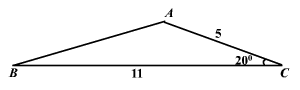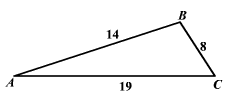# Law of Cosines

The Law of Cosines is used to find the remaining parts of an oblique (non-right) triangle when either the lengths of two sides and the measure of the included angle is known (SAS) or the lengths of the three sides (SSS) are known.  In either of these cases, it is impossible to use the Law of Sines because we cannot set up a solvable proportion.

The Law of Cosines states:

${c}^{2}={a}^{2}+{b}^{2}-2ab\text{\hspace{0.17em}}\text{\hspace{0.17em}}\mathrm{cos}C$ .

This resembles the Pythagorean Theorem except for the third term and if $C$ is a right angle the third term equals $0$ because the cosine of $90°$ is $0$ and we get the Pythagorean Theorem.  So, the Pythagorean Theorem is a special case of the Law of Cosines.

The Law of Cosines can also be stated as

${b}^{2}={a}^{2}+{c}^{2}-2ac\text{\hspace{0.17em}}\text{\hspace{0.17em}}\mathrm{cos}B$ or

${a}^{2}={b}^{2}+{c}^{2}-2bc\text{\hspace{0.17em}}\text{\hspace{0.17em}}\mathrm{cos}A$ .

Example 1: Two Sides and the Included Angle-SAS

Given $a=11,b=5$ and $m\angle C=20°$ . Find the remaining side and angles.${c}^{2}={a}^{2}+{b}^{2}-2ab\text{\hspace{0.17em}}\text{\hspace{0.17em}}\mathrm{cos}C$

$c=\sqrt{{a}^{2}+{b}^{2}-2ab\text{\hspace{0.17em}}\text{\hspace{0.17em}}\mathrm{cos}C}$

$\text{\hspace{0.17em}}\text{\hspace{0.17em}}=\sqrt{{11}^{2}+{5}^{2}-2\left(11\right)\left(5\right)\left(\mathrm{cos}20°\right)}$

$\approx 6.53$

To find the remaining angles, it is easiest to now use the Law of Sines.

$\mathrm{sin}A\approx \frac{11\mathrm{sin}20°}{6.53}$

$A\approx 144.82°$

$\mathrm{sin}B\approx \frac{5\mathrm{sin}20°}{6.53}$

$B\approx 15.2°$

Note that angle $A$ is opposite to the longest side and the triangle is not a right triangle. So, when you take the inverse you need to consider the obtuse angle whose sine is $\frac{11\mathrm{sin}\left(20°\right)}{6.53}\approx 0.5761$ .

Example 2: Three Sides-SSS

Given $a=8,b=19$ and $c=14$ .  Find the measures of the angles.It is best to find the angle opposite the longest side first.  In this case, that is side $b$ .

$\mathrm{cos}B=\frac{{b}^{2}-{a}^{2}-{c}^{2}}{-2ac}=\frac{{19}^{2}-{8}^{2}-{14}^{2}}{-2\left(8\right)\left(14\right)}\approx -0.45089$

Since $\mathrm{cos}B$ is negative, we know that $B$ is an obtuse angle.

$B\approx 116.80°$

Since $B$ is an obtuse angle and a triangle has at most one obtuse angle, we know that angle $A$ and angle $C$ are both acute.

To find the other two angles, it is simplest to use the Law of Sines.

$\frac{a}{\mathrm{sin}A}=\frac{b}{\mathrm{sin}B}=\frac{c}{\mathrm{sin}C}$

$\frac{8}{\mathrm{sin}A}\approx \frac{19}{\mathrm{sin}116.80°}\approx \frac{14}{\mathrm{sin}C}$

$\mathrm{sin}A\approx \frac{8\mathrm{sin}116.80°}{19}$

$A\approx 22.08°$

$\mathrm{sin}C\approx \frac{14\mathrm{sin}116.80°}{19}$

$C\approx 41.12°$